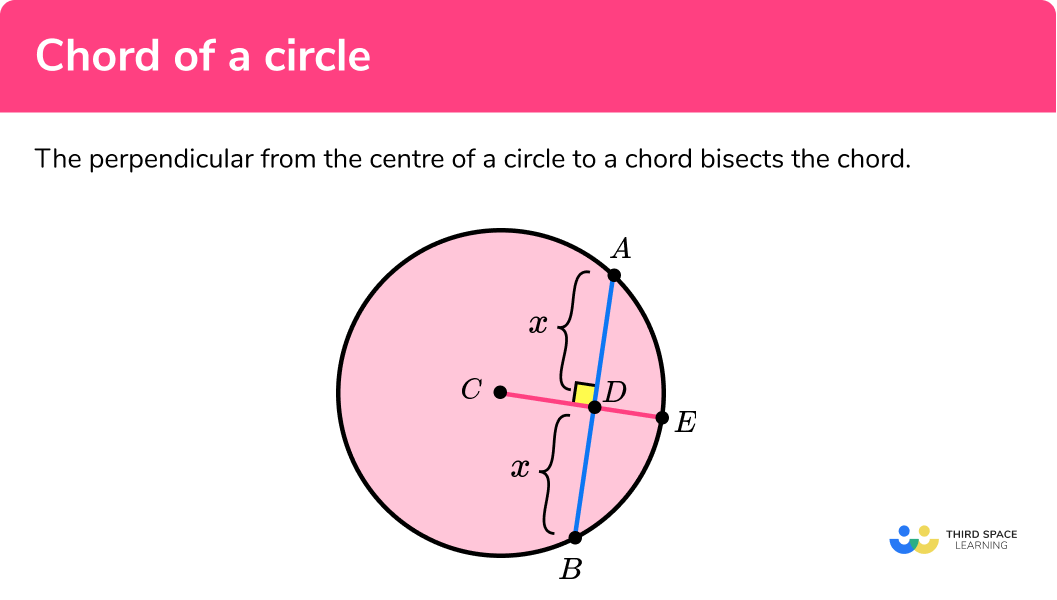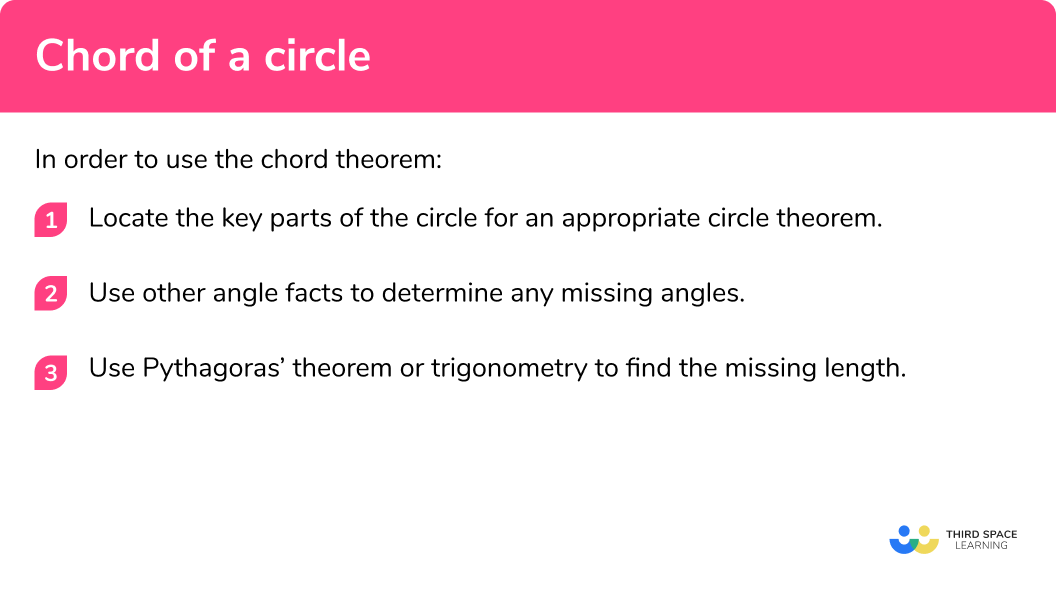# Chord Of A Circle

Here we will learn about circle theorems involving chords of a circle, including its application, proof, and using it to solve more difficult problems.

There are also circle theorem worksheets based on Edexcel, AQA and OCR exam questions, along with further guidance on where to go next if you’re still stuck.

## What is the chord of a circle?

The chord of a circle is a straight line that connects two points on the circumference of a circle. The longest chord in a circle is the diameter of the circle.

The perpendicular from the centre of a circle to a chord bisects the chord (splits the chord into two equal parts).

In the diagram above, AB is a chord and CE is a radius. Here we can see that the radius CE bisects the chord AB at 90 degrees and the lengths AD = BD = x .

### What is the chord of a circle?### Key parts of a circle

Below is a diagram showing the key parts of a circle for this theorem:

• The diameter of the circle is the width of a circle, through the centre. The endpoints of the diameter lie on the circumference of the circle. The diameter is twice the length of the radius of the circle.
• An arc is a part of the circumference.
• The major segment is the larger part of a circle when it is enclosed by a chord and the major arc.
• The minor segment is the smaller part of a circle when it is cut by a chord and the minor arc.
• The radius of a circle is the distance between the centre of the circle and the circumference of the circle.

Subtended angles

An angle within a circle is created by two chords meeting at a point on the circumference. The diagrams below show the inscribed angle subtended by arc AC from point B for two different circles.

Top tip: The word subtend is used a lot within circle theorems so make sure you know what it means.

### Proving that the perpendicular bisector from the centre of a circle to a chord bisects the chord

To be able to prove this theorem, you do not need to know any other circle theorem. You just need to be confident with angles in a triangle and congruence.

## How to find missing lengths using chords

In order to find missing angles or the length of a chord:

1. Locate the key parts of the circle for an appropriate circle theorem.
2. Use other angle facts to determine any missing angles.
3. Use Pythagoras’ Theorem or Trigonometry to find the missing length.

### How to find missing lengths using chordsTangent of a circle is one of 7 circle theorems you will need to know. You may find it helpful to start with our main circle theorems page and then look in detail at the rest.

## Chord of a circle examples

### Example 1: cosine ratio

Below is a circle with centre C . Points A, B, C, and D are on the circumference of the circle. The chord AB is perpendicular to the line CD at the point E . The line AE is 5cm and angle ADE = 71° . Calculate the length of the line BC correct to 1 decimal place.

1. Locate the key parts of the circle for an appropriate circle theorem.

Here we have:

• CD is a diameter
• AB is a chord, perpendicular to CD
• The angle ADE = 71°
• The angle BEC = 90°
• The line AE = 5cm
• The line BC = x

2Use other angle facts to determine other necessary angles.

As angles in the same segment are equal, angle ADE is equal to angle ABC so angle ABC = 71° . Also, as the perpendicular from the centre of a circle to a chord bisects the chord, the line BE is equal to AE , so BE = 5cm .

3Use Pythagoras’ theorem or trigonometry to find the missing length.

To calculate the length of the chord BC , we need to use trigonometry as we know one side length and two angles where one angle is 90° .

As we know the side adjacent to the angle and we want to calculate the hypotenuse, we need to use \cos(\theta)=\frac{A}{H}  with H as the subject.

H=\frac{A}{\cos(\theta)}

x=\frac{5}{\cos(71)}

x=15.4cm (1dp)

### Example 2: sine ratio

Below is a circle with centre O . A, B, C, and D lie on the circumference. AC and BD are perpendicular lines. Calculate the length of AE , correct to 1 decimal place.

Locate the key parts of the circle for an appropriate circle theorem.

Use other angle facts to determine other necessary angles.

Use Pythagoras’ theorem or trigonometry to find the missing length.

### Example 3: tangent ratio

Below is a circle with centre O . A, B, C, and D lie on the circumference. AC and BD are perpendicular lines. FG is a tangent at the point B and is parallel to the line AC . Calculate the length of BE , correct to 1 decimal place.

Locate the key parts of the circle for an appropriate circle theorem.

Use other angle facts to determine other necessary angles.

Use Pythagoras’ theorem or trigonometry to find the missing length.

### Example 4: Pythagoras theorem

A, B, C, and D are points on the circle with centre O . The radius OA = 6cm and the chord AB = 13cm . The chord BD is perpendicular to the diameter AC at E .

OE:OC = 1:1 . Calculate the length of BE marked x .

Locate the key parts of the circle for an appropriate circle theorem.

Use other angle facts to determine other necessary angles.

Use Pythagoras’ theorem or trigonometry to find the missing length.

### Example 5: isosceles triangle

A circle with centre O has the perpendicular diameters AC and BD . Use the information in the diagram to calculate the value of x .

Locate the key parts of the circle for an appropriate circle theorem.

Use other angle facts to determine other necessary angles.

Use Pythagoras’ theorem or trigonometry to find the missing length.

### Example 6: semicircle

The diagram below shows a semicircle. The line BF is a chord that is perpendicular to the diameter. Calculate the length of the diameter AD .

Locate the key parts of the circle for an appropriate circle theorem.

Use other angle facts to determine other necessary angles.

Use Pythagoras theorem or trigonometry to find the missing length.

### Common misconceptions

• Pythagoras’ theorem missing side

The missing side is calculated by incorrectly adding the square of the hypotenuse and a shorter side, or subtracting the square of the shorter sides.

• Incorrect trigonometric function

The incorrect trigonometric function is used and so the side or angle being calculated is incorrect. This also includes the inverse trigonometric functions.

• Incorrect assumption of isosceles triangles

The intersection of the diameter and the chord at 90 degrees can be very close to the centre and so the two lengths coming from the point of intersection to the radius are assumed to be equal, but they aren’t.

### Practice chord of a circle questions

1. A, B, C and D are points on a circle with centre O . BD is the diameter and AC is a chord that is perpendicular to the diameter at E . BE = 2cm and CDE = 30^{\circ} . Calculate the length of x , the distance between C and E .4cm2\sqrt{3}\frac{\sqrt(3)}{6}2cm• AE = x
• BAE = 30^{\circ} (angles in the same segment are equal)
• x=\frac{2}{\tan(30)}=2\sqrt{3}

2. Below is a circle with centre O . AC is a chord, perpendicular to the diameter BD . Use the information in the diagram to calculate the value of x .8.39cm (2dp)6.43cm (2dp)15.56cm (2dp)13.05cm (2dp)• AEB = 90^{\circ} ( AC is perpendicular to BD )
• BAE = 40^{\circ} (angle in a semicircle is 90^{\circ} so BAD=90^{\circ} )
• ABD = 50^{\circ} (angles in a triangle total 180^{\circ} )

3. The circle with centre O has a tangent EF at the point C . The chord BD is parallel to the tangent, intersecting the diameter AC at G . The angle BCF = 51^{\circ} and CG = 3cm . Calculate the length of CD .0.26cm (2dp)2.43cm (2dp)4.77cm (2dp)3.86cm (2dp)• CBG = 51^{\circ} (alternate angles in parallel lines are equal)
• BC = CD = x ( BCD is an isosceles triangle)

4. The line AC is a diameter through the centre of the circle O . BD is perpendicular to AC at E . The radius OA = 10cm and DE = 7cm .
OE=\frac{3}{5}OC . Calculate the length of AB .14.39cm (2dp)12.21cm (2dp)17.46cm (2dp)9.22cm (2dp)• BEA = 90^{\circ} ( AC is perpendicular to BD )
• BE = DE = 7cm ( BD is bisected by the diameter)
• OE=\frac{3}{5}\times{10}=6
• AE = 10 + 6 = 16cm
• x=\sqrt{7^{2}+16^{2}}
• x=17.46cm (2dp)

5. The points A, B, C, and D lie on the circumference of the circle with centre O . The length of BC = 6cm and AC and BD are perpendicular to one another. Calculate the length of OD .\frac{1}{3}cm3cm\frac{\sqrt{2}}{6}cm3\sqrt{2}cm• OC = OD = x
• BOC = 90^{\circ} ( AC and BD are perpendicular)
•where M is the midpoint of BC .
• x=\frac{3}{\sin(45)}=3\sqrt{2}

6. The semicircle has a diameter AD . The chord BF is perpendicular to the diameter at B . BGE = 99^{\circ} and AG = 9cm . AC:CB = 8:3 . Calculate the radius of the semicircle.8.89cm (2dp)6.46cm (2dp)1.02cm (2dp)41.33cm (2dp)• ABF = 90^{\circ} ( BF is perpendicular to AD)</li> <li> BGA = 180 - 99 = 81^{\circ} (angles on a straight line total 180^{\circ} )
• AB = 9sin(81)=8.89cm (2dp)
• AC=\frac{8}{11}AB
• AC = 6.46cm (2dp)

### Chord of a circle GCSE questions

1. Below is a triangle inscribed in a circle with centre O . The line BC is parallel to the tangent DE . BG = 6.2cm . Calculate the length of the line AB .(3 marks)

ABC = 55^{\circ} (alternate segment theorem)

(1)

AGB = 90^{\circ} ( BC is perpendicular to AF )

(1)

(1)

2. (a) The circle below has centre O. The diameter BD is perpendicular to the chord AC at point E. CE = 5.4cm and angle AB = 8.1cm. Calculate the length of the line BE.(b) Calculate the size of the angle ABE. Write your answer to 1 decimal place.

(5 marks)

(a)
AE = CE = 5.4cm

(1)

BE=\sqrt{8.1^{2}-5.4^{2}}

(1)

BE = 6.04cm (2dp)

(1)

(b)
ABE=\sin^{-1}(\frac{5.4}{8.1})

(1)

ABE = 41.8^{\circ} (1dp)

(1)

3. The diagram below shows a circle with the following information:

• Centre O
• AC is a straight line through B
• DH = 3cm
• BH = 8cmCalculate the length of BC , labelled x .

(5 marks)

CDA = 90^{\circ} as the tangent meets the radius at 90^{\circ} and AHB = 90^{\circ} as BF is parallel to CE

(1)

AH = BH = 8cm
AB=\sqrt{8^{2}+8^{2}}=8\sqrt{2}

(1)

Scale factor of enlargement: 11\div8=1.375

(1)

AC=8\sqrt{2}\times{1.375}=11\sqrt{2}

(1)

11\sqrt{2}-8\sqrt{2}=3\sqrt{2}cm

(1)

## Learning checklist

You have now learned how to:

• Apply and prove the standard circle theorems concerning angles, radii, tangents and chords, and use them to prove related results

## Still stuck?

Prepare your KS4 students for maths GCSEs success with Third Space Learning. Weekly online one to one GCSE maths revision lessons delivered by expert maths tutors.

Find out more about our GCSE maths tuition programme.